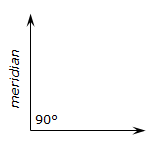## 1. General

Point position can be expressed in relative or absolute terms.

### a. Relative position

A relative position is the either the (a) length and direction, or, (b) latitude and departure between two points.
Give the adjusted traverse of Figure F-1:Figure F-1 Adjusted Loop Traverse

Lengths and directions around a traverse define the relative locations of successive traverse points.

Point B is 472.72 feet from point A at a bearing of S 68°05'27"W.

Point C is 216.12 feet from point B at a bearing of N 19°46'15"W.

In terms of latitudes and departures:

Point B is 176.39 feet South and 438.57 feet West of point A.

Point C is 203.38 feet North and 73.10 feet West of point B.

Where is point C relative to point A? Because the two points are not directly connected on the traverse, it requires a little more computing.

Lat A to C: [-472.72 ft x cos(68°05'27") + 216.12 ft x cos(19°46'15")] = +26.99 ft

Dep A to C: [-472.72 ft x sin(68°05'27") - 216.12 ft x sin(19°46'15")] = -511.68 ft

Point C is 28.99 ft North and 511.68 ft West of point A. We could compute the lats and deps through point D instead of point B - the distances from point A to point C would be the same.

### b. Absolute Position

An absolute position is a distance from a datum. In the case of a traverse point, two horizontal lines serve as the data. One line corresponds with the meridian, the other is perpendicular to it, Figure F-2.Figure F-2 Horizontal Datum

The meridional line is called either the Y or North (N) axis; the other the X or East (E) axis.

A point position is expressed as a coordinate pair are represent perpendicular distances from the two axes.

For example:In terms of an X and Y system, Figure F-3, the coordinates of point P are X=225.64' and Y=320.95' Figure F-3 X and Y SystemIn terms of an North and East system, Figure F-4, the coordinates of point P are E=225.64' and N=320.95' Figure F-4 North and East System